# Richard Sprague

My personal website

# Read Bristle Health Raw Data

Created: 2022-07-14 ; Updated: 2022-08-26

The oral microbiome testing company, Bristle, gives me a web page table of all the microbes they found in my mouth. Can I turn that web page table into a more useful R dataframe?

The data is kept in a format with columns for `genus`, `species`, and `relative abundance`. That’s easy to read straight from Excel format.

``````library(tidyverse)

raw_bristle_data %>% transmute(genus,species,abundance=`relative abundance`/100) %>%
group_by(genus) %>% summarize(sum=sum(abundance)) %>%
arrange(desc(sum)) %>% slice_max(order_by=sum, prop=.5) %>% # summarize(total=sum(sum))# %>%
ggplot(aes(x=reorder(genus,-sum), y=sum)) + geom_col() +
theme(axis.text.x=element_text(angle = 90, vjust = 0.5)) +
labs(y="Abundance (%)", x = "Genus", title = "Bristle Health Result")``````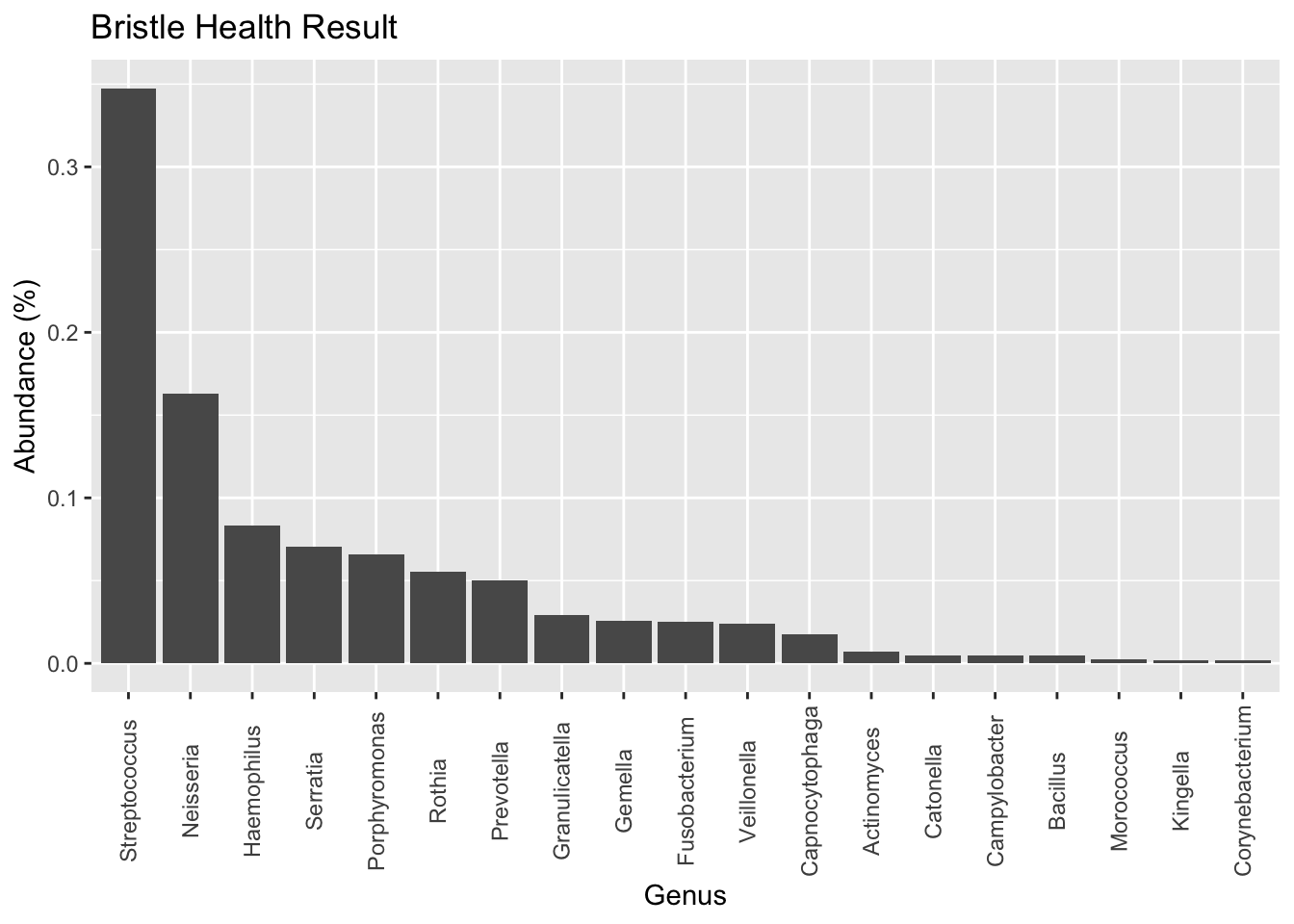## Make a Tree Map

``````if(!require("treemap")) install.packages("treemap")

raw_bristle_data %>% transmute(genus,species,abundance=`relative abundance`/100) %>%
treemap::treemap(dtf=., index = c("genus","species"),
vSize="abundance",
title = "My Mouth Microbes")``````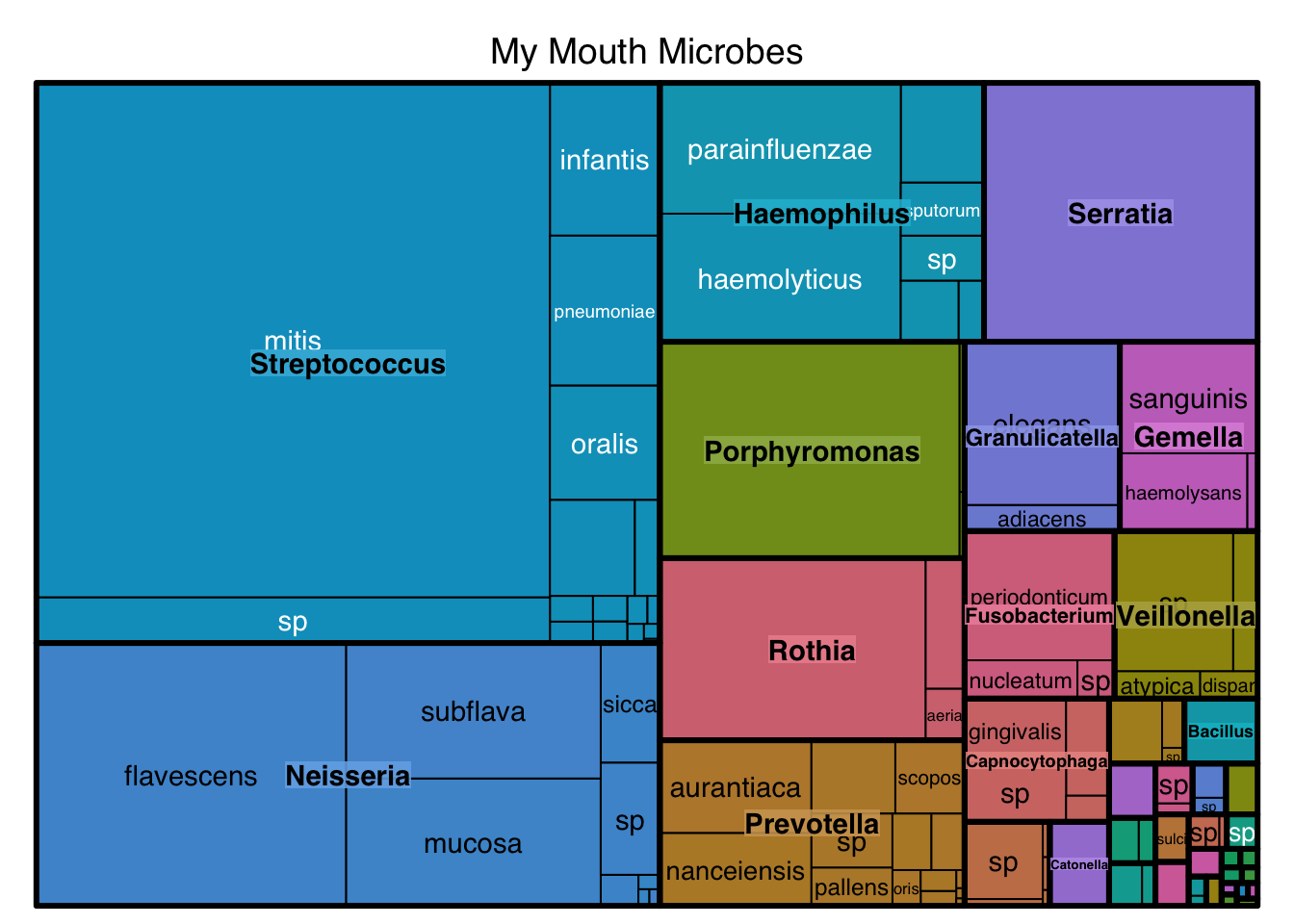## 16S Samples

Make a dataframe of all uBiome samples (genus level only). Requires some special packages.

``````library(actino)
library(phyloseq)
library(psmr)  # use library function instead.

options(scipen = 999)

mouth.genus <- subset_samples(sprague.genus.norm, Site == "mouth")

mouth_df <- mhg_df_just_taxa(mouth.genus,"Streptococcus") %>% select(Date,Label,abundance)

bristle_genus <- raw_bristle_data %>% select(genus) %>% pull(genus) %>% unique()

ub_labels <- mhg_df_just_taxa(mouth.genus,"Streptococcus") %>% pull(Label)

ub_mouth_genus_df_abundances <- sapply(bristle_genus[c(1:16,18:31,34,37)],function(x) {mhg_df_just_taxa(mouth.genus,x) %>% pull(abundance)/1000000}) %>% as.data.frame()

ub_mouth_genus_df <- cbind(ub_labels,ub_mouth_genus_df_abundances)``````

## Plot 16S Data

``````ub_mouth_genus_of_sample <- function(sample_number = 1, genus_df=ub_mouth_genus_df) {
.rownames <- genus_df[sample_number,-1] %>% t() %>% as.data.frame() %>% rownames()
return(tibble(genus = .rownames,abundance = genus_df[sample_number,-1] %>% t() %>% as.vector() ))
}

ub_mouth_genus_of_sample(1)  %>%
slice_max(order_by=abundance, prop=.5) %>% # summarize(total=sum(sum))# %>%
ggplot(aes(x=reorder(genus,-abundance), y=abundance)) + geom_col() +
theme(axis.text.x=element_text(angle = 90, vjust = 0.5)) +
labs(y="Abundance (%)", x = "Genus", title = "16S Sample Result")``````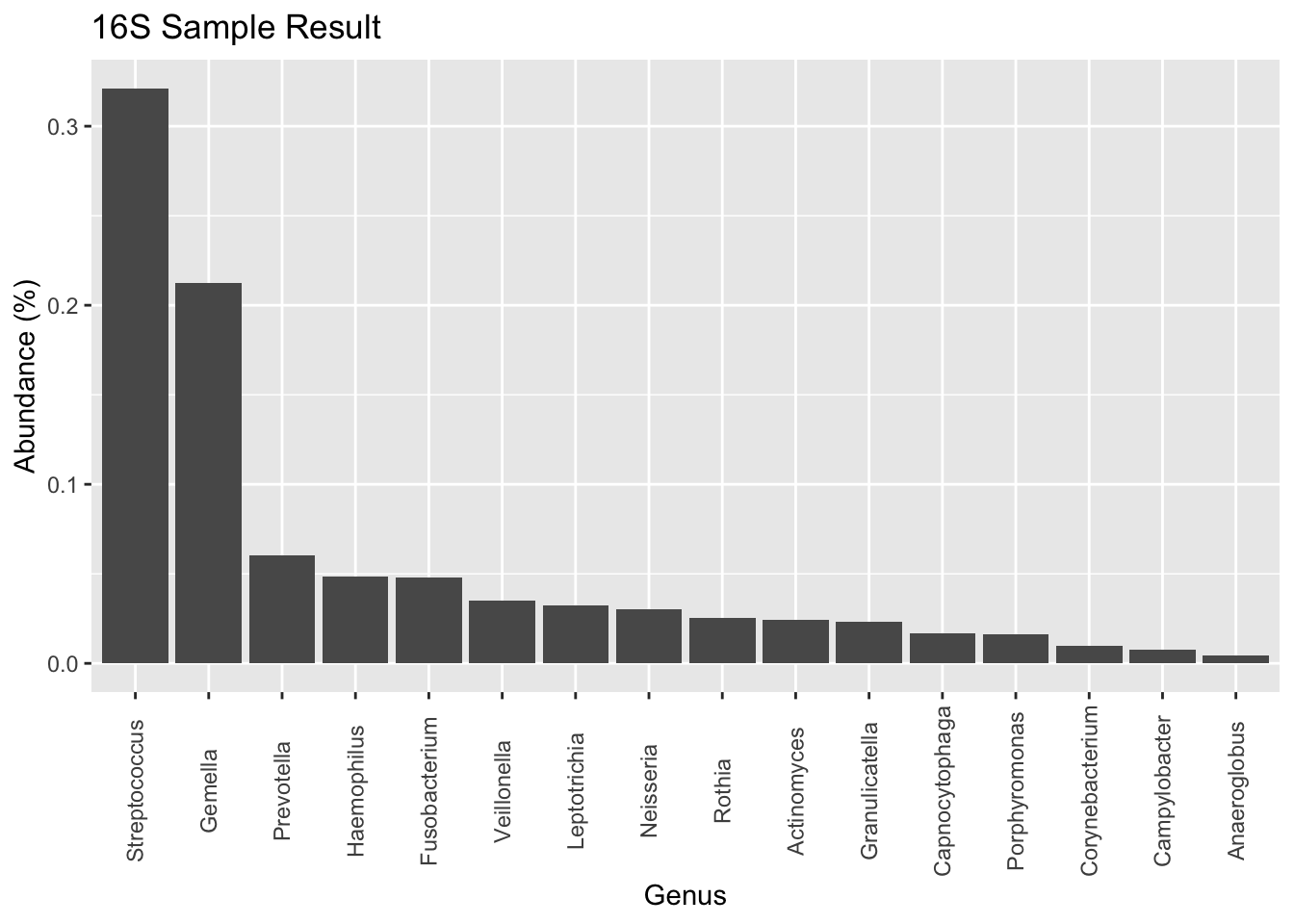## Tree Map of 16S Data

``````ub_mouth_genus_of_sample(75) %>%
treemap::treemap(dtf=., index = c("genus"),
vSize="abundance",
title = "My Mouth Microbes (Before Dentist)")``````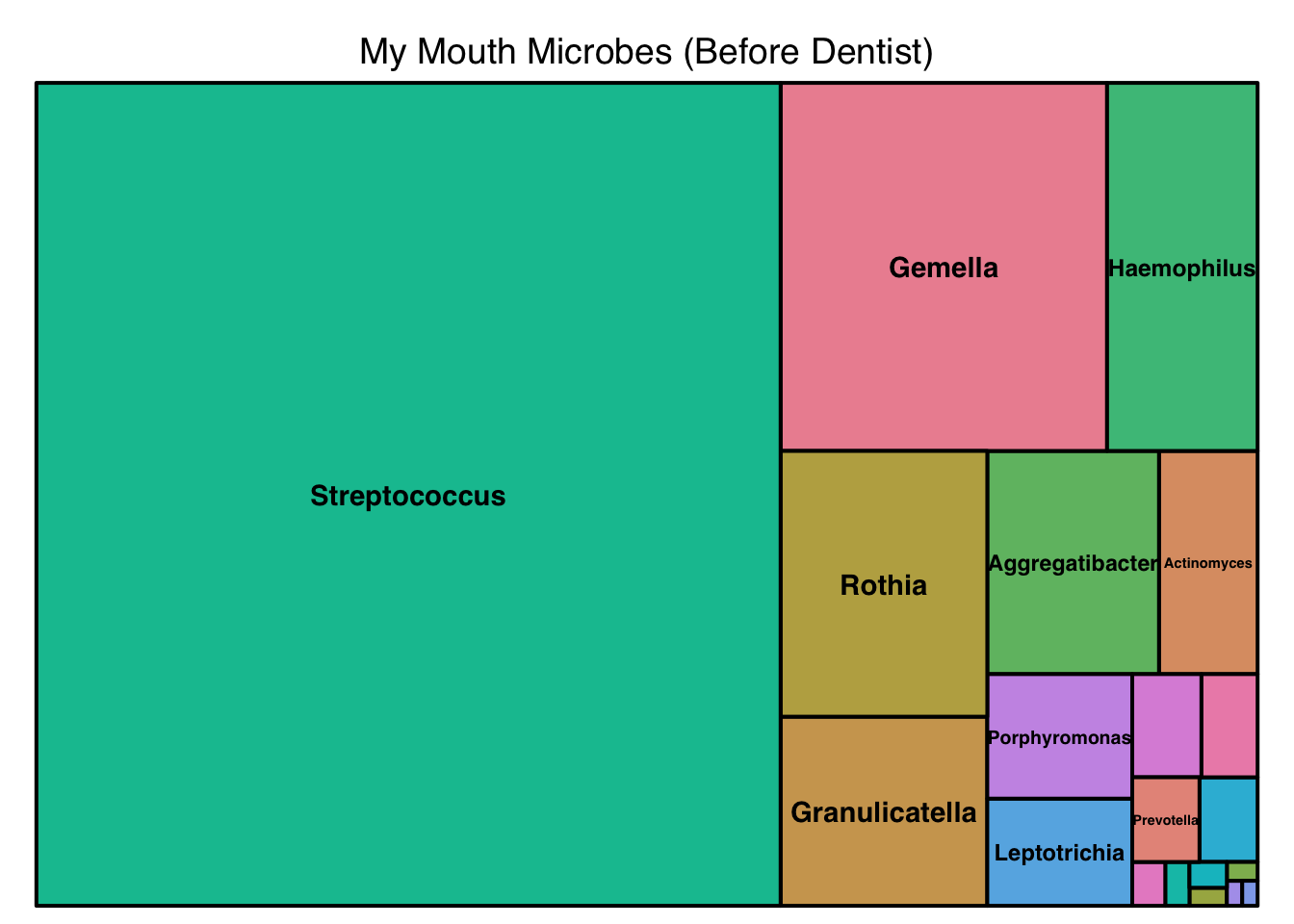``````ub_mouth_genus_of_sample(76) %>%
treemap::treemap(dtf=., index = c("genus"),
vSize="abundance",
title = "My Mouth Microbes (After Dentist)")``````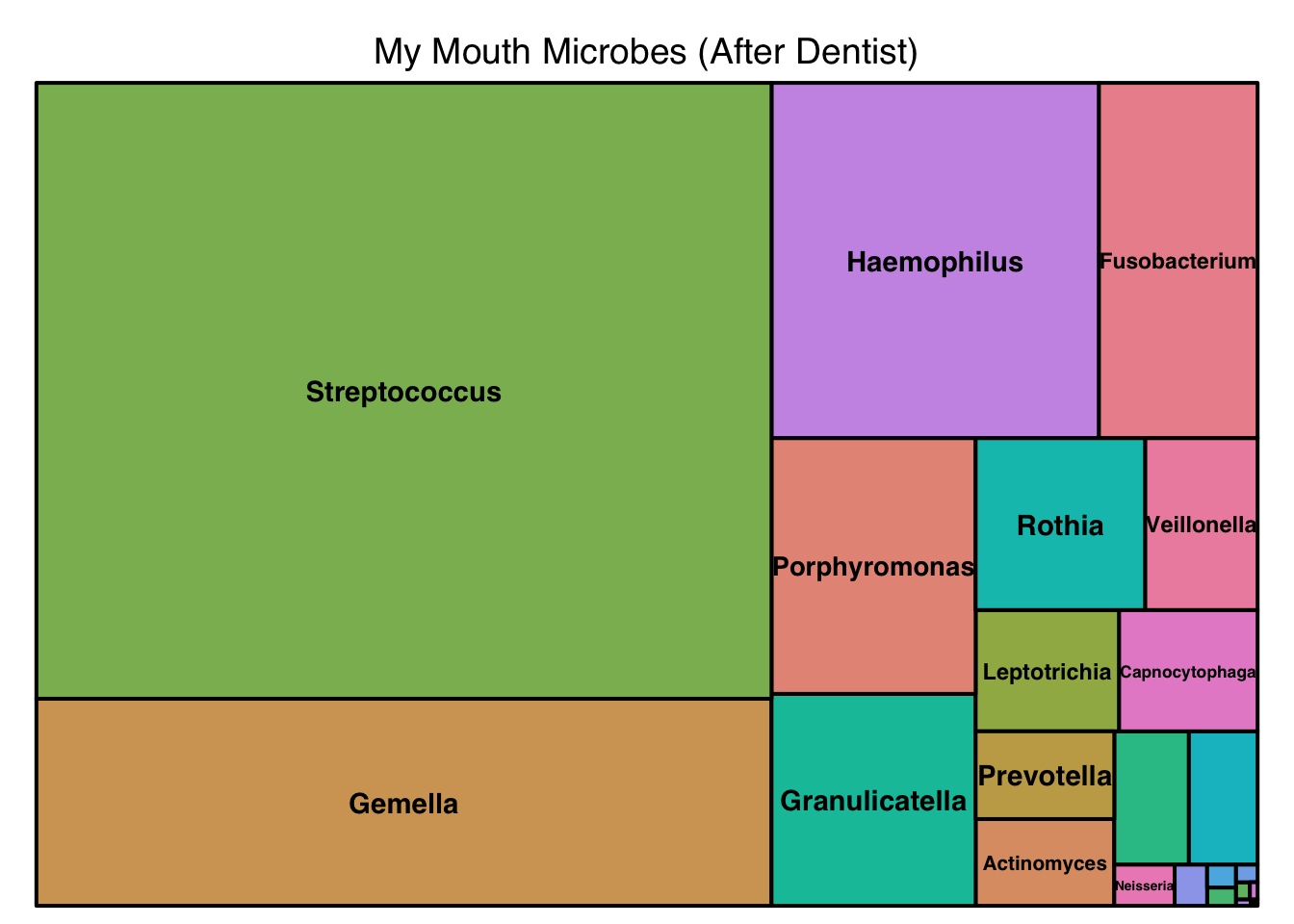P.S. You can try this yourself using my R Shiny Web app

Also please see some of my recent Bristle Reports